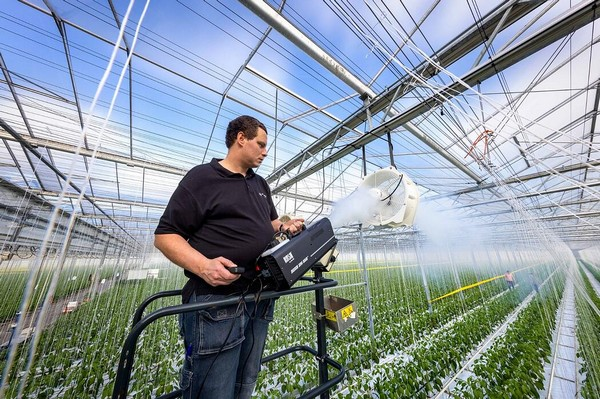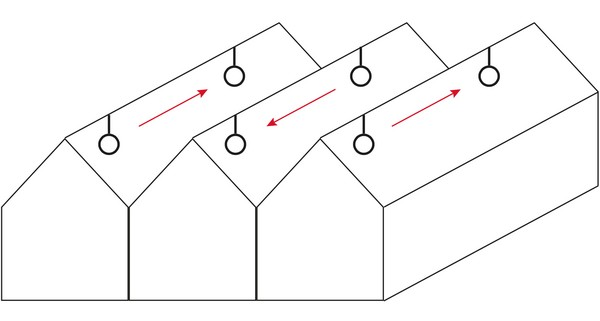# How do I determine the number of fans in a greenhouse?

To calculate the number of fans in a greenhouse, it is important to have three basic factors available. With these basic factors, Vostermans Ventilation can calculate the number of fans step by step.Basic factors
Greenhouse dimensions: Start by calculating the volume of the entire greenhouse and make a note of the dimensions.

Circulation rate: The circulation rate is equal to how many times per hour the air is circulated in a greenhouse. In practice, we often see that a circulation rate of 2 or 3 is chosen. In some situations, a circulation rate of 12 or higher is chosen. The ideal circulation rate depends on the situation on-site, and the crop.

Fan specification: Finally, it is important to know how many m3 of air the fan moves per hour, and what the maximum recommended usable throw is. Vostermans' Greenhouse Fans are divided into 3 lines: the 5,000, 7,000 and 8,500 m3/h versions. You can find this information on the lid of the fan motor, in the brochure, or the datasheet.

Current situation
In the current situation, our assumptions are based on a greenhouse of 160,000 m3. If we multiply this by the circulation rate of 3, we know that the circulation fans have to move 480,000 m3 of air per hour.

Step 1: Number of fans to achieve the circulation rate
To know how many m3 of air must be circulated each hour, we can simply divide this by the number of m3 that a fan moves per hour. Depending on your wishes, you can choose a fan from the 5,000, 7,000 or 8,500 m3/h line. In this case, we have assumed an ideal situation with ideal greenhouse sizes and suspension points at any desired location. We have included these variables in steps 2 and 3. We then arrive at the following number of fans:

4,850 m3/h: 99 fans
6,500 m3/h: 74 fans
8,700 m3/h: 55 fans

Step 2: Distance between fans
To prevent fans being located outside of each other's airflow, we recommend that you do not exceed the recommended throw. We have verified this recommended maximum usable throw in practice, with smoke tests. In practical terms, this means that with hanging options every 4 meters, a fan with a recommended throw of 35 meters will be suspended at a distance of 32 meters.Step 3: Greenhouse dimensions
Finally, the fans should be evenly distributed along the length of the greenhouse. In practice, the length of the greenhouse is often not divisible by the fan distance calculated in step 2. In this case, the fans are hung even closer together to obtain the right distribution in the greenhouse, and to avoid exceeding the maximum recommended throw.Vostermans Ventilation
https://www.vostermans.com/ventilation/greenhouse

Publication date: